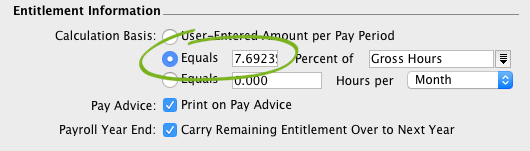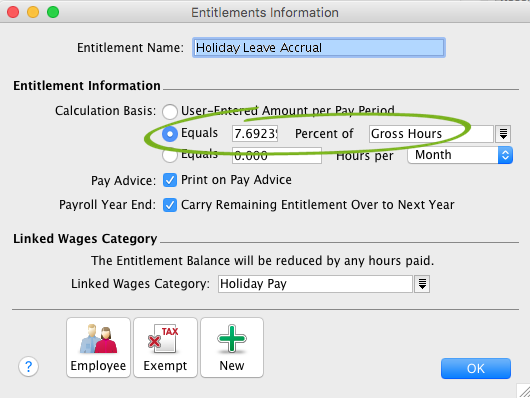##### Child pages
• Calculating payroll accrual percentages (Australia only)

AccountEdge Pro and Network Edition, Australia only

When setting up leave accruals, if you select the Equals [x] Percent of option as the calculation basis, you might need to calculate the percentage.The National Employment Standards specify that as a minimum, full-time and part-time employees get 4 weeks of annual leave, based on their ordinary hours of work. Also, the minimum personal leave entitlement is 10 days per year for full-time employees, or pro rata of 10 days for part-time employees, depending on their hours of work.

If your employees are entitled to...use this percentage...
4 weeks annual leave per year (or pro-rata for part-time employees)7.6923%
10 days personal leave per year (or pro-rata for part-time employees)3.8462%

If you're setting up a different type of leave, or an employee is entitled to more than the minimum leave entitlement, you'll need to calculate the entitlement percentage.

## Need help working it out?

Use this simple calculator to work out your entitlement percentage. Just enter the employee's standard weekly hours and yearly leave entitlement (in weeks) and you're done.

Remember, the yearly leave entitlement is what the employee is entitled to, not necessarily what they'll accrue. E.g a part timer might be entitled to 4 weeks annual leave, but might only accrue 2 weeks based on their hours worked.

Total hours worked per week =

Total weeks of leave per year =

Entitlement = %

Here are the details and formula behind this calculation:

Calculation details

### Calculation details

To work out the entitlement percentage you need to know:

• the total yearly entitlement (number of weeks leave entitled to in a year, eg. 6 weeks annual leave, 3 weeks personal leave, etc.)
• the total hours worked in a year (hours worked per week x 52)

Whatever type of leave you're setting up, use this formula to calculate the entitlement percentage:

Entitlement percentage = (Total yearly entitlement / Total hours worked per year) x 100

Example 1: Annual leave entitlement

Say your employees work 38 hours a week and are entitled to 5 weeks annual leave per year.

To calculate the entitlement percentage:

Total yearly entitlement = 38 hours per week × 5 weeks = 190
Total hours worked per year = 38 hours per week × 52 weeks = 1976

(190/1976) x 100 = 9.6154%

Example 2: Personal leave entitlement

Say your employees work 7.6 hours a day and are entitled to 15 days (3 weeks) personal leave per year.

To calculate the accrual percentage:

Total yearly entitlement = 38 hours per week × 3 weeks = 114
Total hours worked per year = 38 hours × 52 weeks = 1976

(114/1976) x 100 = 5.7692%

## Examples

To calculate the percentage rate for 20 days leave per year

Let's say an employee works 38 hours per 5-day week (7.6 hours a day) and they're entitled to 20 days (4 weeks) leave per year.

The entitlement percentage formula is:

(Total annual entitlement hours / total annual work hours) X 100 = %

• Total annual entitlement hours = 20 days X 7.6 hours a day = 152 hours
• Total annual work hours = 38 hours per week X 52 weeks = 1976 hours
• So the entitlement percentage is (152 / 1976) X 100 = 7.6923%

So if an employee is entitled to 20 days holiday leave per year, the percentage to use in their entitlement category is 7.6923%This means that for every hour the employee works, they will accrue .076923 hours of holiday leave.

To calculate the percentage rate for fewer than 20 days per year

### Example: 8 days personal leave entitlement

Let's say an employee works 38 hours per 5-day week (7.6 hours a day) and they're entitled to 8 days personal leave per year.

The entitlement percentage formula is:

(Total annual entitlement hours / total annual work hours) X 100 = %

• Total annual entitlement hours = 8 days X 7.6 hours a day = 60.8 hours
• Total annual work hours = 38 hours per week X 52 weeks = 1976 hours
• The entitlement percentage is (60.8 / 1976) X 100 = 3.0769 %

### Example: 10 days personal leave entitlement

Let's say an employee works 40 hours per 5-day week (8 hours a day) and they're entitled to 10 days personal leave per year.

The entitlement percentage formula is:

(Total annual entitlement hours / total annual work hours) X 100 = %

• Total annual entitlement hours = 10 days X 8 hours a day = 80 hours
• Total annual work hours = 40 hours per week X 52 weeks = 2080 hours
• The entitlement percentage is (80 / 2080) X 100 = 3.8461 %